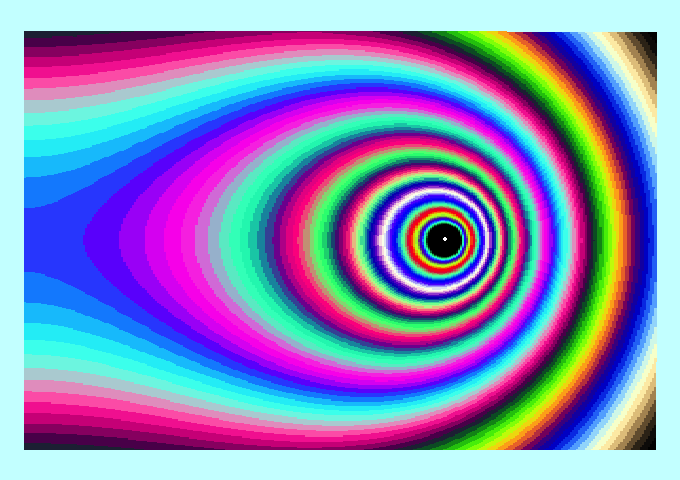# Template:LSU CT99CommonTheme1Awhite

In §102 of a book titled, The Theory of the Potential, MacMillan (1958; originally, 1930) derives an analytic expression for the gravitational potential of a uniform, infinitesimally thin, circular "hoop" of radius, $~a$. Throughout our related discussions, we generally will refer to this additional Key Equation from MacMillan as providing an expression for the,
Gravitational Potential in the Thin Ring (TR) Approximation$~\Phi_\mathrm{TR}(\varpi,z)$ $~=$ $~-\biggl[ \frac{2GM}{\pi } \biggr]\frac{K(k)}{\sqrt{(\varpi+a)^2 + z^2}}$ $\mathrm{where:}~~~k \equiv \{4\varpi a/[ (\varpi+a)^2 + z^2]\}^{1 / 2}$